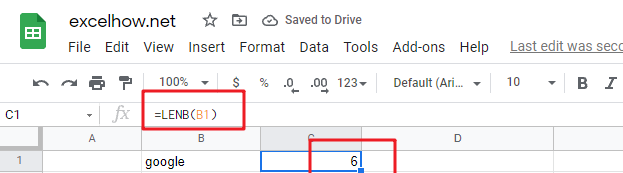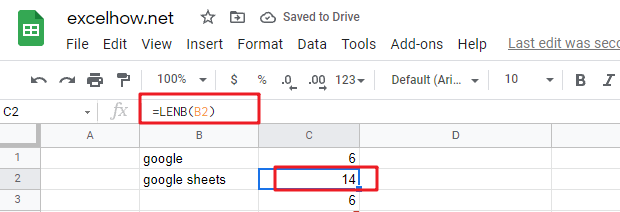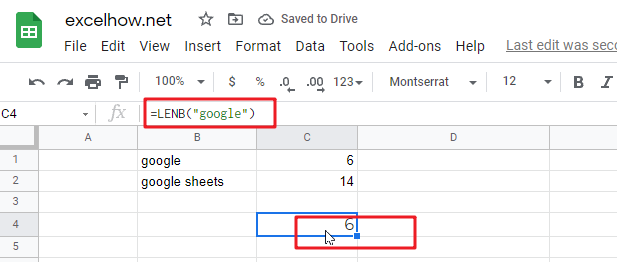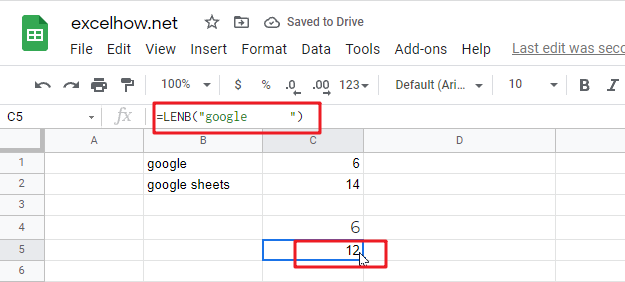# ExcelHow

This post will guide you how to use Google Sheets LENB function with syntax and examples.

## Description

The Google Sheets LENB function returns the length of a text string in bytes.

The LENB function can be used to count the number of bytes of a given text string including space or nonprint characters in google sheets. If a text string is a double byte character set, for example, Japanese, Chinese and Korean, each character should be counted as 2 bytes.

The LENB function is a build-in function in Google Sheets and it is categorized as a Text Function.

## Syntax

The syntax of the LENB function is as below:

= LENB (text)

Where the LENB function arguments are:

• Text -This is a required argument. The text string that you want to get the length of.

Note:

• The LENB function only takes one argument. It counts the number of bytes in text string, including space or non-printing characters. If the LENB function returns unexpected values, and you need to make sure that there are no such characters in given text string.
• If text string is an empty string or cell reference to an empty cell, the LENB function returns
• LENB function will also count the characters in numeric value.

## Google Sheets LENB Function Examples

The below examples will show you how to use google sheets LENB Text function to get the length of a text string in bytes.

#1 To get the number of bytes in the B1 cell, just using formula:

`=LENB(B1)`#2 To get the Length of the text string in B2 Cell, just using formula:

`=LENB(B2)`#3 Using LENB function to count the number of bytes in a text string:

`=LENB(“google”)   //it returns 6`#4 count the number of bytes in a text string in which contain a space character:

`=LENB(“google      “)  //it returns 9`The LENB function can be used with the LEFT function and FIND function to extract a substring text to the left of a given text string .

## Related Functions

The Google Sheets FIND function returns the position of the first text string (sub string) within another text string.The syntax of the FIND function is as below:= FIND(find_text, within_text,[start_num]) …
returns a substring (a specified number of the characters) from a text string, starting from the leftmost character. The syntax of the LEFT function is as below:= LEFT(text,[num_chars]) …